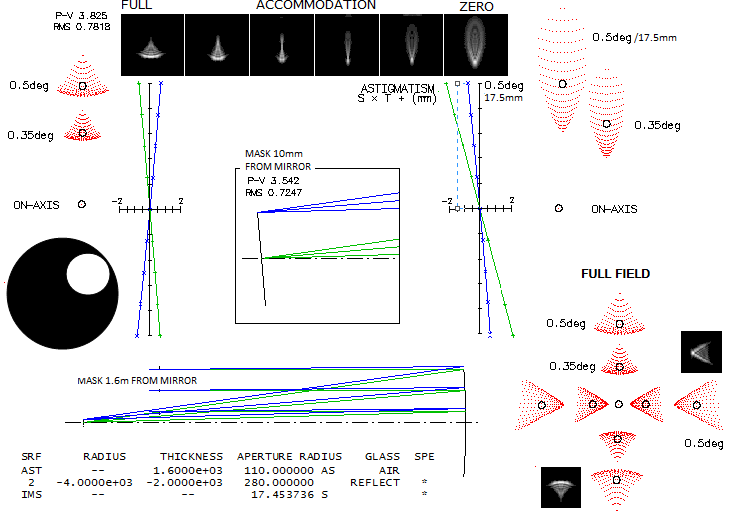telescopeѲptics.net          ▪▪▪▪                                             CONTENTS

# 8. REFLECTING TELESCOPES: Newtonian, two- and three-mirror systems

As one would think, reflecting telescopes use mirror objectives to form the image. They come in a variety of arrangements, from a single mirror objective to those consisting of several mirrors. Most of designs are obstructed, with the secondary mirror in the light path - such are Newtonian and Cassegrain varieties. Unobstructed designs, either with tilted or off-axis elements, enjoy small but steady popularity. Inherent optical quality varies from one design to another, and so do other characteristics, including needed properties of optical surfaces, and sensitivity to miscollimation.

## 8.1. The Newton reflector

The most popular telescope design among amateurs - Newtonian reflector - consists from a single concave primary mirror and a reflecting flat. It is a single optical surface of the primary mirror that creates the image, while the flat merely directs light out to the side, to an accessible observing location. Ideal shape of the primary mirror is paraboloid, which is free from spherical aberration when imaging distant objects. Smaller, longer focus mirrors can be left spherical - which is the easiest to make and test mirror shape - but will have residual amounts of spherical aberration (FIG. 112). Concave mirror also produces off-axis aberrations which are, for distant object and stop at the surface, independent of its conic constant.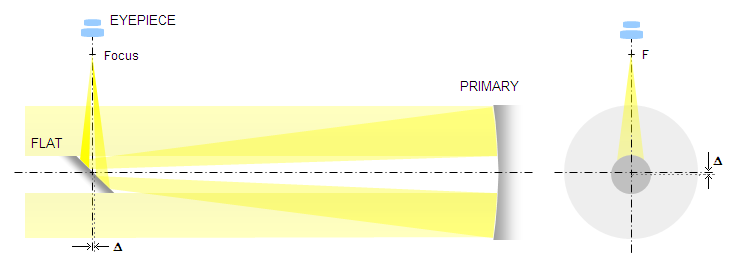FIGURE 112: Newtonian reflector: simple telescope design invented and built by the famous English physicist, mathematician, and astronomer Sir Isaac Newton in 1672. First reflecting telescope was probably built by Niccolò Zucchi in 1616, but Newton was the first to introduce the diagonal flat - a simple solution his predecessors failed to see. Light converges from the concave primary mirror to the flat diagonal mirror, which reflects it out to the side, where is located the eyepiece. The primary can be left spherical with smaller long focus mirrors. Newton's own reflector used 1.3" ~ƒ/5 spherical mirror, since he did not know how to produce paraboloid. Medium and larger diameters mirrors have to be parabolized, due to spherical aberration of a sphere becoming excessive for astronomical use. Fast Newtonian telescopes usually have the flat "offset", i.e. slightly shifted down and forward () to even up field illumination and make collimation easier.

With distortion being zero for the stop at surface, remaining primary aberrations are spherical, coma, astigmatism and field curvature. A quick look at the aberration coefficients gives following wavefront errors for the concave mirror:

lower-order spherical aberration, from Eq. 7,9.1-2 is given for object at infinity by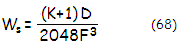as the P-V wavefront error at the best focus (1/4 of the error at paraxial focus), with K being the mirror conic, D the aperture diameter and F the focal ratio. Evidently, the only surface form free from spherical aberration for object at infinity is a paraboloid (K=-1). The RMS wavefront error for primary spherical aberration is smaller than the P-V error by a constant ratio; it is given by ω=W/1.55, or: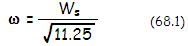In units of the λ=0.00055mm wavelength, the P-V wavefront error is: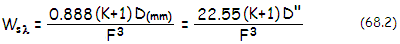for the aperture D in mm, and in inches, respectively, with the corresponding RMS wavefront error ω=/11.25.

Longitudinal and transverse aberrations are as given in 2.1 Spherical aberration. The RMS wavefront error, in units of the wavelength, can be used to calculate the appropriate Strehl ratio from Mahajan's close approximation (Eq. 56). For spherical mirror, it can also be expressed as:

S ~ e-(1.66D/F3)2 ~ 1/e(1.66D/F3)2           (69)

for the natural logarithm base e~2.718, and the aperture diameter D in mm. Taking conventional 0.80 Strehl, or the RMS wavefront error in units of the wavelength ω=1/180 as the maximum acceptable amount of wavefront degradation, sets the appropriate F# limit for spherical mirror at F=(3.55D)1/3 or larger for D in mm, and
F=(90.17D)
1/3 for D in inches. Counting in the effect of central obstruction, the criterion becomes more demanding, as described in 5. Obstruction effects.

From  S~1-(2πω)2, needed F# for a desired Strehl S with spherical mirror is given by F~3.5D1/3/(1-S)1/6, for the aperture diameter D in inches, and F~1.18D1/3/(1-S)1/6 for D in mm (since based on the approximation, it is accurate for Strehl ratios of ~0.9 and higher; for the ratios ~0.8 and lower, it gives increasingly higher F# than the actual value).

For objects close enough that the primary magnification m, defined as one of Eq. 9 parameters, appreciably differs from zero, the P-V wavefront error at the best focus, after substituting for m in terms of the object distance o and mirror focal length ƒ, is W's=-[K+(1-2ψ)2]D/2048F3 (the minus sign indicating the aberration is numerically negative at the best focus location; consequently, it is positive, or overcorrected at paraxial focus), with ψ=ƒ/o being the primary focal length in units of the object distance (the reciprocal of the object distance in units of the mirror focal length). Obviously, the wavefront error is zero if the expression in the brackets is zero, which defines the zero-aberration conic in terms of object distance, for primary spherical aberration, as K=-(1-2ψ)2.

Inversely, object distance in terms of wavefront error induced can be written as (1-2ψ)2=[2048WF3/D]-K. For the wavefront error in units of 550nm wavelength, it is (1-2ψ)2=-[1.126WF3/D]-K. For a selected wavefront error, the right side gives the value of (1-2ψ) squared, which makes finding ψ easy. For instance, for W=0.05 wave in units of 550nm wavelength, D=400mm ƒ/4 paraboloidal mirror (thus F=4 and K=-1), the value of (1-2ψ)2 is 0.99099, thus (1-2ψ)=0.99549 and ψ=(1-0.99549)/2=0.00226. The corresponding distance is ƒ/0.00226=443ƒ=708.9 meters, nearly identical to the value obtained with somewhat rounded off relation given in the section on star testing.

Most Newtonian telescopes nowadays use paraboloidal primary. If well made, its spherical aberration is practically cancelled. However, off-axis aberrations are present, and can be significant - particularly coma. Before addressing full aperture off axis aberrations of a Newtonian, a quick look at the effect of off-axis mask, in a fairly common use with larger instruments of this kind.